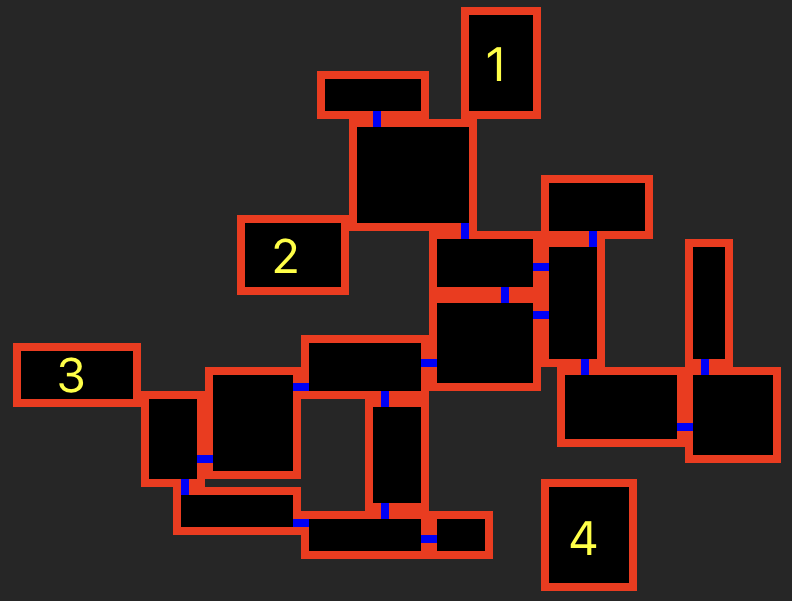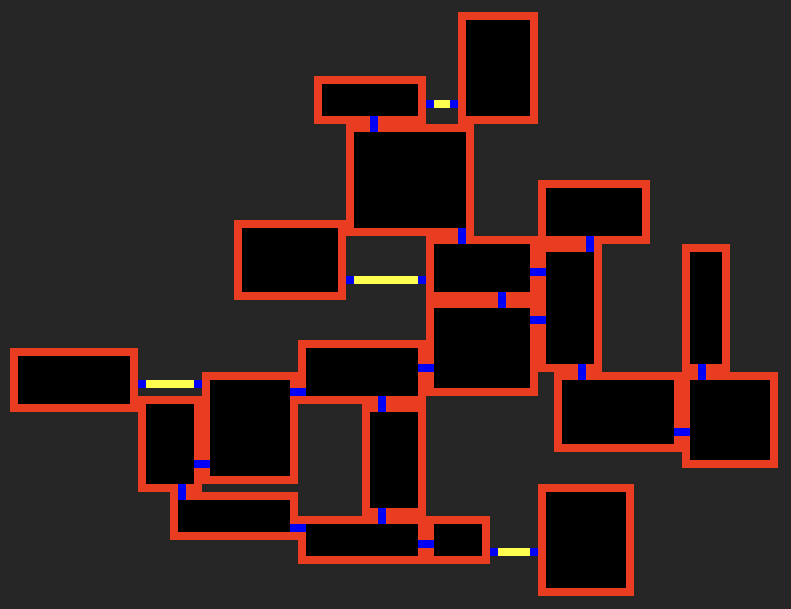# SF Rogue Corridor Generation

This article continues covering the challenges I faced while creating SF Rogue.

## Challenge 4

Now that we have our random rooms generated, that don't overlap and connecting to other rooms, we need to deal with "orphaned" rooms that could not connect due to location within the level.

### The Problem

Firstly it will help to explain the problem we are trying to solve in a bit more detail. As the rooms are created and moved to eliminate overlaps, some rooms will be left with no touching rooms...The above image shows that there are 4 rooms in this level that are not connected, the first 3 do have touching rooms, but not enough space to put a door in, while the fourth room is truly on its own.

### The Solution

I'm sure there are probably a number of solutions to this problem, but the one that came to me was to create an imaginary rectangle thats the height of a corridor (3 tiles) and the width of the whole level. Starting at the bottom edge of the room find other rooms that intersect that rectangle and see if a connection can be made...If no intersecting rooms where found (though obviously there is in this version), keep moving the rectangle up a tile until you've run out of height. If a room still hasn't been found repeat the process vertically.

### The Code

We will continue using the same playground we created in the previous article, which can be found on GitHub.

To get things started we need to add a few `Constants` values, add the following...

``````static let corridorSize = Constraints.tileSize * 3       // The dimension of a corridor, 2 walls and a floor tile
static let floatTileSize = CGFloat(Constraints.tileSize) // A CGFloat version of the tile size, for convenience
``````

Add the following computed properety to `Room` so that we can easily identify the orphaned rooms...

``````public var noDoors: Bool {
return doors.count == 0
}``````

Now in the main `Playground` we can include a function that will control the addition of corridors into the level. Add the following...

``````func findCorridors() {
// Calculate the whole area the rooms are contained in
var levelArea = CGRect.zero
for room in rooms {
levelArea = room.frame.union(levelArea)
}

// Find the rooms that don't have an doors,
// obviously not all levels will contain rooms with no doors
let roomsWithoutDoors = rooms.filter( { \$0.noDoors } )
for room in roomsWithoutDoors {
// Attempt to create a corridor and add it to the array of rooms
if let corridor = room.generateCorridor(levelArea, rooms) {
rooms.append(corridor)
}
}
}``````

Then add a call to `findCorridors()` in the `didMove` function, after the call to `findConnections()` and before the rendering of the rooms...

``````findConnections()
findCorridors()

for room in rooms {``````

Now the Playground will highlight errors as a `Room` doesn't know how to `generateCorridor`. We will start correcting that now, its worth pointing out that a corridor is just a long narrow `Room`. Add the following function to the `Room`

``````    public func generateCorridor(_ levelArea: CGRect, _ rooms: [Room]) -> Room? {
// The unique number of the room if it gets created
let roomNumber = rooms.count

// Try and create a horizontal corridor
if let corridor = generateHorizontalCorridor(levelArea, rooms, roomNumber) {
return corridor
}

// We've not created a horizontal one, so try and create a vertical one
if let corridor = generateVerticalCorridor(levelArea, rooms, roomNumber) {
return corridor
}

return nil
}``````

The `generateHorizontalCorridor` function will control looking for possible rooms and if any are found attempt to create the corridor...

``````func generateHorizontalCorridor(_ levelArea: CGRect, _ rooms: [Room], _ roomNumber: Int) -> Room? {
// Calculate the imaginary rectangle that extends the width of the level
// Place it at the bottom edge of the room
var horizontalExtension =  CGRect( x: Int(levelArea.minX),
y: Int(frame.minY) ,
width:  Int(levelArea.width),
height: Constants.Constraints.corridorSize)
repeat {
// Move the rectangle up a tile (first time this allows for missing the wall
horizontalExtension = horizontalExtension.offsetBy(dx: 0, dy: Constants.Constraints.floatTileSize)

// Find any rooms that intersect this area, that are to the left of this room
// and sort them so that the nearest one is the first in the results
let intersectingLeftRooms = rooms.filter(
{ \$0.frame.intersects(horizontalExtension) &&
\$0.leftEdge < leftEdge
}
).sorted { \$0.leftEdge > \$1.leftEdge }

// If we have a room, see if we can create the corridor, if it is return it
if let possibleRoom = intersectingLeftRooms.first,
let corridor = createHorizontalCorridor(horizontalExtension, possibleRoom, .left, roomNumber) {
return corridor
}

// Find any rooms that intersect this area, that are to the right of this room
// and sort them so that the nearest one is the first in the results
let intersectingRightRooms = rooms.filter(
{ \$0.frame.intersects(horizontalExtension) &&
\$0.rightEdge > rightEdge
}
).sorted { \$0.rightEdge < \$1.rightEdge }
// If we have a room, see if we can create the corridor, if it is return it
if let possibleRoom = intersectingRightRooms.first,
let corridor = createHorizontalCorridor(horizontalExtension, possibleRoom, .right, roomNumber)  {
return corridor
}

// We've not created a corridor, if we have space to move the imaginary
// rectangle up then loop back round and try again

} while Int(horizontalExtension.maxY) < topEdge

return nil
}``````

Creating the corridor is a case of checking that the intersection can fit at least one floor tile, calculating the room dimensions, creating the `Room` and adding a door at each end. This means we need an additional `init` for the `Room` class that takes dimensions rather than cols/rows, it will also give us the chance to draw a different colour for corridor floors...

``````public init(number: Int, rect: CGRect) {
self.number = number

self.cols = Int(rect.width) / Constants.Constraints.tileSize
self.rows = Int(rect.height) / Constants.Constraints.tileSize

self.node = SKShapeNode(rectOf: rect.size)
self.node.lineWidth = 0
self.node.name = "room\(self.number)"

self.node.position = CGPoint(x: rect.midX, y: rect.midY)
// Create a basic node using the size reduced by 2 tile sizes in each direction
let floor =  SKShapeNode(rectOf: CGSize(width: Int(rect.width) - (Constants.Constraints.tileSize * 2),
height: Int(rect.height) - (Constants.Constraints.tileSize * 2)))
floor.lineWidth = 0
floor.fillColor = .yellow
}``````

Now that we have the new constructor, we can add the function to generate the corridor, we already have functions that will add the doors...

``````func createHorizontalCorridor(_ horizontalExtension: CGRect, _ possibleRoom: Room, _ direction: Constants.DoorWall, _ roomNumber: Int) -> Room? {
// Calculate the intersection with the room and check that the area can actually fit a corridor
let intersection = possibleRoom.frame.intersection(horizontalExtension)
if Int(intersection.height) == Constants.Constraints.corridorSize, Int(intersection.width) >= Constants.Constraints.tileSize {
// Calculate the dimensions based on the direction the corridor is going
let corridorRect = direction == .left
? CGRect(x: possibleRoom.rightEdge,
y: Int(horizontalExtension.minY),
width: abs(leftEdge - possibleRoom.rightEdge),
height: Constants.Constraints.corridorSize)
: CGRect(x: rightEdge,
y: Int(horizontalExtension.minY),
width: abs(possibleRoom.leftEdge - rightEdge),
height: Constants.Constraints.corridorSize)

// Create the room
let corridorRoom = Room(number: roomNumber, rect: corridorRect)

corridorRoom.createConnectingDoor(toRoom: self, basedOnWall:  direction == .left ? .right : .left)
corridorRoom.createConnectingDoor(toRoom: possibleRoom, basedOnWall: direction == .left ? .left : .right)
return corridorRoom
}

return nil
}
``````

The code for generating vertical corridors is very similar, just looking in a different direction. Rather than repeat this here, take a look at the completed `Playground` on GitHub. Theres no additional code to render the corridors as they are just rooms and will get processed along with the other rooms.

## Challenge 4 Completed

Orphaned rooms are now connected with corridors! All of the challenges have now been completed, (well sort of read the Closing Comments below).Whilst the code in these articles explain the processes I went through, it doesn't show all the code I used to generate the levels in my game. As you run this code you will notice that there are times where "groups" of rooms are orphaned and will not be connected using the code supplied. There are few additional hoops that my app jumps through to ensure all rooms can be reached and I don't want to give away all of my code. But the challenges that I've left for you to solve are really not that complicated, if you get stuck feel free to drop me some questions.

There are also other improvements that could be made

• Corridors with bends in them
• Random walls that are checked first, so that there is more of a mix of vertical and horizontal corridors
• Picking the corridor direction based on proximity rather than direction